# Geometry and Measure - Short Problems

The collections below contain short problems about Geometry and Measures.

You may also be interested in our longer problems on Geometry and Measures.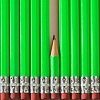### Angles, Polygons and Geometrical Proof Short Problems

A collection of short problems on Angles, Polygons and Geometrical Proof.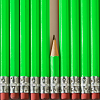### Construction - Short Problems

A collection of short problems on construction and loci.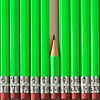### 3D Shapes - Short Problems

A collection of short problems on 3D shapes.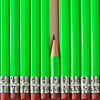### Perimeter, Area and Volume Short Problems

A collection of short problems on area and volume.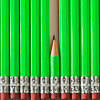### Transformations - Short Problems

A collection of short problems on transformations.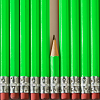### Vectors - Short Problems

A collection of short problems on vectors.### Pythagoras' Theorem and Trigonometry - Short Problems

A collection of short problems on Pythagoras's Theorem and Trigonometry.